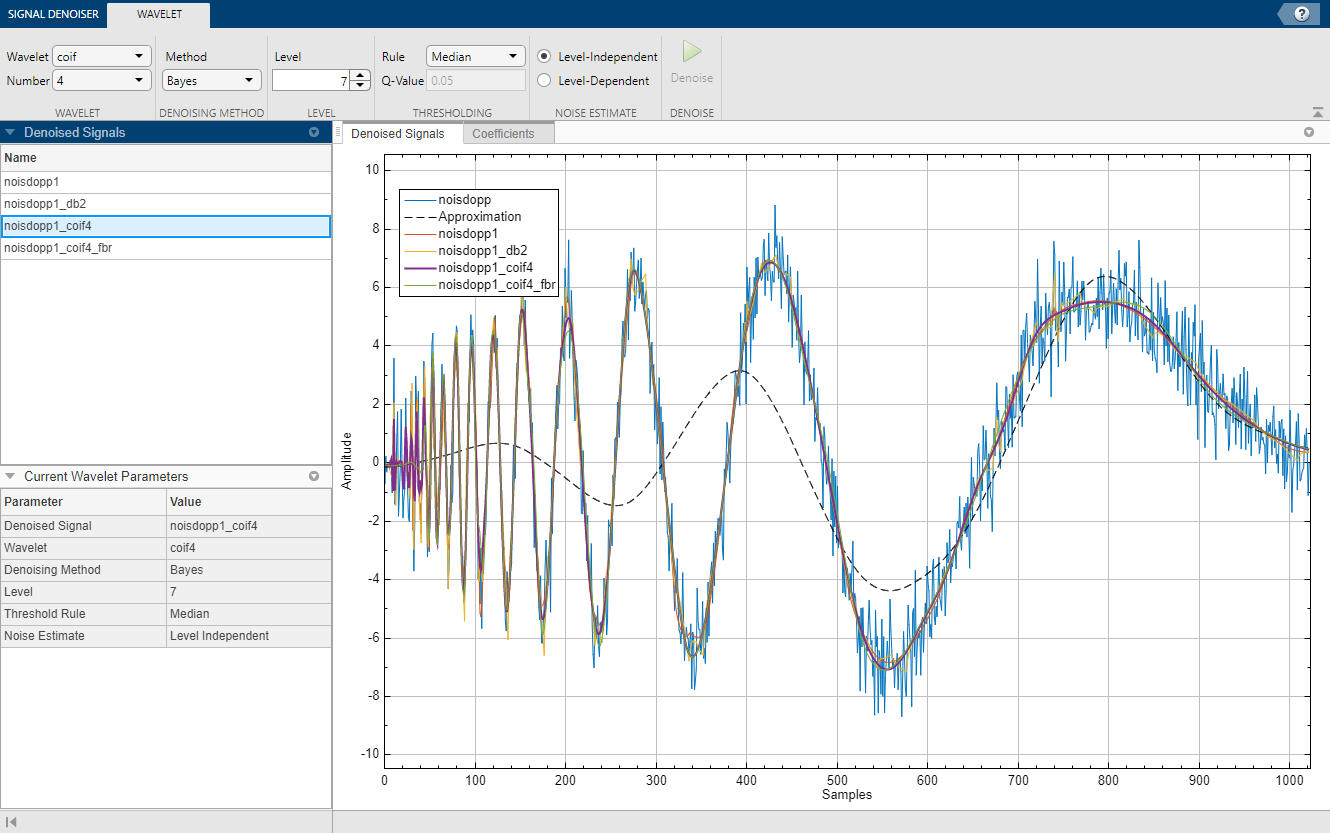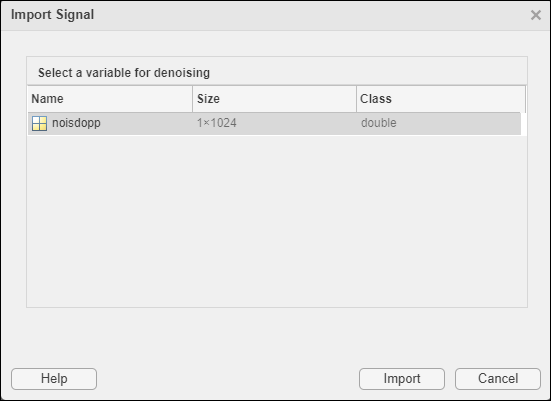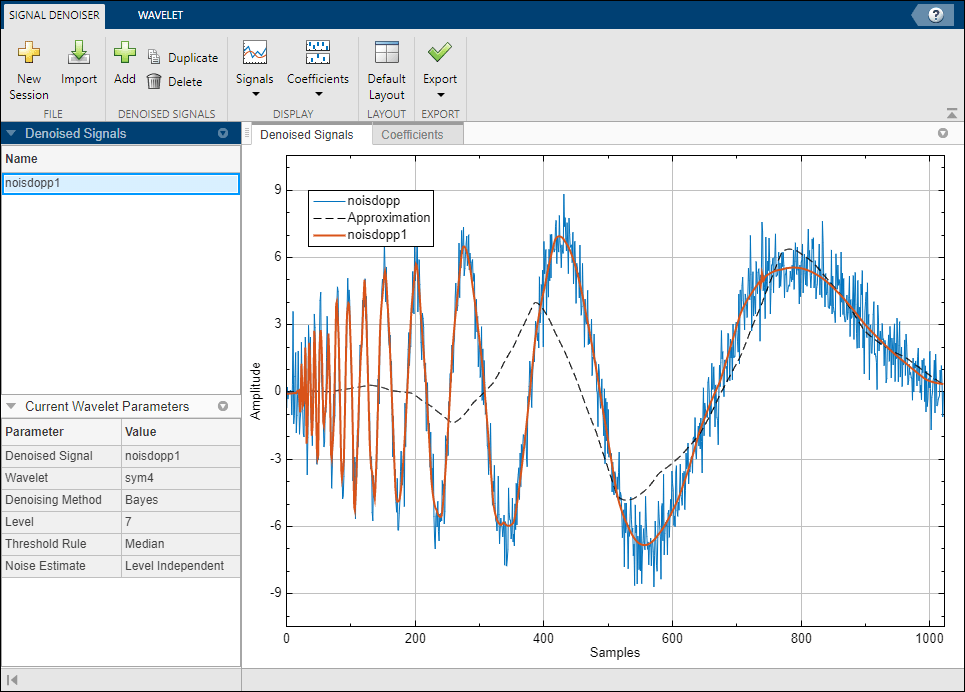# Wavelet Signal Denoiser

Visualize and denoise time series data

## Description

The Wavelet Signal Denoiser app is an interactive tool for visualizing and denoising real-valued 1-D signals and comparing results. With the app, you can:

• Access all the signals in the MATLAB® workspace.

• Easily adjust default parameters and apply different denoising techniques.

• Visualize and compare results.

• Export denoised signals to your workspace.

• Recreate the denoised signal in your workspace by generating a MATLAB script.

The Wavelet Signal Denoiser app provides a way to work with multiple versions of denoised data simultaneously.

A typical workflow for denoising a signal and comparing results using the app is:

1. Start the app and load a 1-D signal from the MATLAB workspace. The app provides an initial denoised version of your data using default parameters.

2. Adjust the denoising parameters and produce multiple versions of the denoised signal.

3. Compare results and export the desired denoised signal to your workspace.

4. To apply the same denoising parameters to other signals in your workspace, generate a MATLAB script and modify it as you see fit.## Open the Wavelet Signal Denoiser App

• MATLAB Toolstrip: On the Apps tab, under Signal Processing and Communications, click Wavelet Signal Denoiser.

• MATLAB command prompt: Enter `waveletSignalDenoiser`.

## Examples

expand all

This example shows how to denoise a 1-D signal using the app default settings.

`load noisdopp`

Start the Wavelet Signal Denoiser app by choosing it from the Apps tab on the MATLAB® Toolstrip. You can also start the app by typing `waveletSignalDenoiser` at the MATLAB command prompt.

Load the noisy Doppler signal from the workspace into the app by clicking Load Signal in the toolstrip. From the list of workspace variables that can be loaded into the app, select `noisdopp` and click OK.The app displays the original signal, `noisdopp`, the denoised signal, `noisdopp1`, and the coarse scale approximation, `Approximation`.To toggle what plots are visible, you can:

• Click Signals ▼ in the toolstrip and use the drop-down menu to toggle the visibility of the original and approximation plots.

• Click individual signals in the plot legend.

## Parameters

Wavelet family used to denoise the signal, specified as one of the following:

• `sym` — Symlets

• `bior` — Biorthogonal spline wavelets

• `coif` — Coiflets

• `db` — Daubechies wavelets

• `fk` — Fejér-Korovkin wavelets

Denoising method to apply, specified as one of the following:

• `Bayes` — Empirical Bayes

• `BlockJS` — Block James-Stein

• `FDR` — False Discovery Rate

• `Minimax` — Minimax Estimation

• `SURE` — Stein's Unbiased Risk Estimate

• `UniversalThreshold` — Universal Threshold $\sqrt{2\mathrm{ln}\left(\text{length}\left(x\right)\right)}.$

Thresholding rule to use. The possible rules you can specify for different denoising methods are as follows:

• Block James-Stein — `James-Stein`

• Empirical Bayes — `Median`, `Mean`, `Soft`, `Hard`

• False Discovery Rate — `Hard`

• Minimax Estimation — `Soft`, `Hard`

• Stein's Unbiased Risk Estimate — `Soft`, `Hard`

• Universal Threshold —`Soft`, `Hard`

## Tips

To denoise more than one signal simultaneously, you can run multiple instances of the Wavelet Signal Denoiser app.